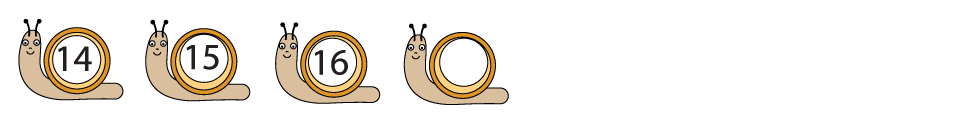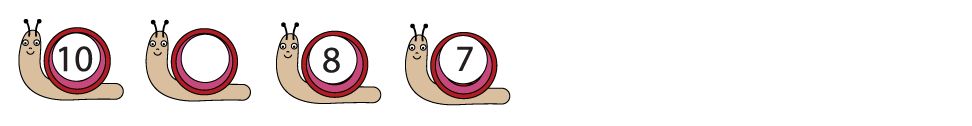A sequence is a pattern which is always the same.

Look at this example:The pattern here is to add 1 to each number.

16 + 1 is 17, so the next number is 17.

Look at this next example:The pattern is to take 1 away from each number.

10 - 1 is 9, so the missing number is 9.

Now, let's start the activities!